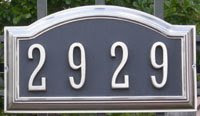## Friday, November 21, 2008

### 2929

2929 = 29 x 101.

2929 has two representations as a sum of two squares: 2929 = 152 + 522 = 252 + 482.

2929 is the hypotenuse of two primitive Pythagorean triples: 29292 = 15602 + 24792 = 16792 + 24002.

2929 is a square-free number because its prime decomposition contains no repeated factors.

2929 is a divisor of 10014 - 1.2929 Entertainment is a media company with interests ranging from movie production to video games.